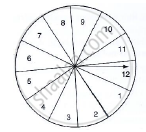Share

# A Game of Chance Consists of Spinning an Arrow Which is Equally Likely to Come to Rest Pointing to One of the Number, 1, 2, 3, ..., 12 as Shown in Fig. Below. What is the Probability that It Will Point To A Number Which is Multiple of 3? - CBSE Class 10 - Mathematics

ConceptProbability - A Theoretical Approach

#### Question

A game of chance consists of spinning an arrow which is equally likely to come to rest
pointing to one of the number, 1, 2, 3, ..., 12 as shown in Fig. below. What is the
probability that it will point to a number which is multiple of 3?#### Solution

Total no. of possible outcomes = 12 {1, 2, 3,…., 12}

Let E ⟶ event of pointing at a no. multiple of 3

No. favourable outcomes = 4 {3, 6, 9, 12}

P(E) ="No.of favorable outcomes"/"Total no.of possible outcomes"

P(E) =4/12 =1/3

Is there an error in this question or solution?

#### Video TutorialsVIEW ALL 

Solution A Game of Chance Consists of Spinning an Arrow Which is Equally Likely to Come to Rest Pointing to One of the Number, 1, 2, 3, ..., 12 as Shown in Fig. Below. What is the Probability that It Will Point To A Number Which is Multiple of 3? Concept: Probability - A Theoretical Approach.
S